### Descriptive Statistics

Suppose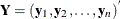is the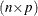matrix of complete data, which might not be fully observed,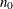is the number of observations fully observed, and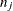is the number of observations with observed values for variable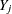.

With complete cases, the sample mean vector is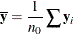and the CSSCP matrix is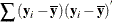where each summation is over the fully observed observations.

The sample covariance matrix is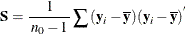and is an unbiased estimate of the covariance matrix.

The correlation matrix, which contains the Pearson product-moment correlations of the variables, is derived by scaling the corresponding covariance matrix: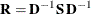where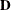is a diagonal matrix whose diagonal elements are the square roots of the diagonal elements of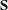.

With available cases, the corrected sum of squares for variableis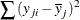where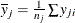is the sample mean and each summation is over observations with observed values for variable.

The variance is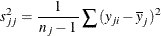The correlations for available cases contain pairwise correlations for each pair of variables. Each correlation is computed from all observations that have nonmissing values for the corresponding pair of variables.